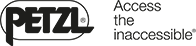Fall factor and impact force - theory - Petzl Belgique• Dealers
• Customer service
•Search

Fall factor and impact force - theory

Fall factor and impact force are two important concepts in the physics of climbing falls. To understand a climbing fall, it is important to recall a basic law of physics: when an object falls, it stores energy.

Warnings

• Read the technical notice before viewing the following techniques.
• It is important to fully understand the information provided in the technical notice before using this complementary information
• Mastering these techniques requires training.
• Consult a professional before attempting to perform these techniques on your own.

Impact force

During fall arrest, this energy is dissipated by elongation of the rope, displacement of the belayer, the climber's body... Energy is transmitted to the belay chain in the form of force. This is the impact force. For the climber, it's the impact experienced during fall arrest.

We are often interested in the impact force transmitted to the climber, the belayer, and the redirect point.

This value relates to all of the important factors in energy absorption: rope elongation, belayer displacement, the climber's body, rope sliding through the device...

The impact force indicated on a rope corresponds to the maximum force measured on a metal mass (a climber) in the standard test conditions (see Impact force-standards).

Theoretical fall factor

The fall factor is often used to quantify the severity of a climbing fall.

It can have a value between 0 and 2 in climbing.Fth =

Fall length

Rope length

Fth = theoretical fall factor

Fall length = length of the climber's fall

Rope length = length of rope between belayer and climber

The fall factor is the ratio of fall length to rope length.

In climbing the severity of the fall dœs not depend on the fall length, as the longer the rope, the more energy it can absorb.In these two cases, the severity of the fall increases. The free fall length is the same. There is the same amount of energy to absorb, but the system is less dynamic.

Case 1

rope length = 10 m, fall length = 4 m so fall factor = 4/10 = 0.4.

The rope length is significant, so the absorption capacity is significant. The severity is low, so the impact force is low.

Case 2

rope length = 2 m, fall length = 4 m so fall factor = 4/2 = 2.

The rope length is short, so the absorption capacity is low. The severity is significant.

In theory, the higher the fall factor, the higher the forces generated. The concept of severity as a function of fall factor is useful only with a dynamic rope. The longer the rope, the more energy it can absorb. The fall factor model is rather simplistic, as it dœs not take into account important factors such as rope drag, type of belay device, belayer displacement... In the following chapters, we will see the impact of some of these factors.

Actual fall factor

The theoretical fall factor dœs not take into account the rope friction against the rock and in quickdraws. This friction prevents the rope from stretching over its entire length. Thus, only a part of the rope (solid line) will absorb the energy of the fall: this is called effective rope length. It is therefore useful to talk about the actual fall factor. It is clear that if a climber dœs not take the necessary steps to avoid rope drag, the actual fall factor can quickly increase. In this case, the fall will be more severe for the climber.Fr =

Fall length

Effective rope length

Fa = actual fall factor

Fall length = length of the climber's fall

Effective rope length = actual length of rope in play